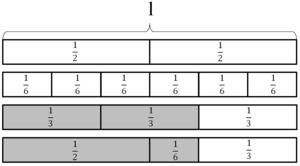Egyptian Fractions

Alignments to Content Standards: 5.NF.A.1

Ancient Egyptians used unit fractions, such as $\frac{1}{2}$ and $\frac{1}{3}$, to represent all fractions. For example, they might write the number $\frac{2}{3}$ as $\frac{1}{2} + \frac{1}{6}$.We often think of $\frac{2}{3}$ as $\frac{1}{3}+\frac{1}{3}$, but the ancient Egyptians would not write it this way because they didn't use the same unit fraction twice.

1. Write each of the following Egyptian fractions as a single fraction:
1. $\frac{1}{2} + \frac{1}{3}$,
2. $\frac{1}{2}+ \frac{1}{3} + \frac{1}{5}$,
3. $\frac{1}{4} + \frac{1}{5} + \frac{1}{12}$.

2. How might the ancient Egyptians have writen the fraction we write as $\frac{3}{4}$?

IM Commentary

One goal of this task is to help students develop comfort and ease with adding fractions with unlike denominators. Another goal is to help them develop fraction number sense by having students decompose fractions. Because the Egyptians represented fractions differently than we do, it can also help students understand that there can be many ways of representing the same number. This helps prepare them for writing algebraic expressions in 6th grade. This task is an instructional task; the teacher may wish to supplement part (b) of the question in two directions:

1. As is indicated in the solution, there are many ways to write $\frac{3}{4}$ as a sum of unit fractions and student work may naturally lead to a discussion of this issue.
2. Students might be prompted to try to express some other fractions, such as $\frac{2}{5}$ or $\frac{3}{5}$ as sums of unit fractions.

For historical accuracy, it should be noted that the ancient Egyptians had special hieroglyphic symbols for the two fractions $\frac{2}{3}$ and $\frac{3}{4}$ considered in this problem. In general, however, they used tables in order to break down fractions into sums of unit fractions.

One issue which comes up in the solution is that there are apparently many ways to write an Egyptian fraction. This leads to an interesting question: how do you find the "best'' Egyptian fraction representing a given fraction? Here a reasonable interpretation of "best'' would be one which has the smallest number of unit fractions. This problem will be addressed in a second task which falls under the high school algebra standards.

Solution

1. For the Egyptian fraction $\frac{1}{2} + \frac{1}{3}$, a common denominator would be $6$ since $6$ is divisible by both $2$ and $3$. Converting to this common denominator we find

\begin{align} \frac{1}{2} + \frac{1}{3} &= \frac{3 \times 1}{3 \times 2} + \frac{2 \times 1}{2 \times 3} \\ &= \frac{3}{6} + \frac{2}{6} \\ &= \frac{5}{6}. \end{align}

For $\frac{1}{2} + \frac{1}{3} + \frac{1}{5}$ we could use what we have just found, namely that $\frac{1}{2} + \frac{1}{3} = \frac{5}{6}$. To add $\frac{5}{6}$ and $\frac{1}{5}$ we can use $5 \times 6 = 30$ as a common denominator:

\begin{align} \frac{1}{2} + \frac{1}{3} + \frac{1}{5} &= \frac{5}{6} + \frac{1}{5} \\ &= \frac{5 \times 5}{5 \times 6} + \frac{6 \times 1}{6 \times 5} \\ &= \frac{25 + 6}{30}\\ &= \frac{31}{30}. \end{align}

For $\frac{1}{4}$, $\frac{1}{5}$, and $\frac{1}{12}$ note that $12$ is divisible by $4$ so we can look for a common denominator of $\frac{1}{5}$ and $\frac{1}{12}$ and this will also work with $\frac{1}{4}$. For $\frac{1}{5}$ and $\frac{1}{12}$ we can use $5 \times 12$ as a common denominator:

\begin{align} \frac{1}{4} + \frac{1}{5} + \frac{1}{12} &= \frac{15 \times 1}{15 \times 4} + \frac{12 \times 1}{12 \times 5} + \frac{5 \times 1}{5 \times 12} \\ &= \frac{15}{60} + \frac{12}{60} + \frac{5}{60} \\ &= \frac{15+12+5}{60} \\ &= \frac{32}{60}. \end{align}
As a parenthetical note, this gives an example where even though we found the least common denominator to perform the addition, the resulting fraction is not in reduced form: the reduced form is $\frac{8}{15}$.

2. To write $\frac{3}{4}$ as an Egyptian fraction, we might notice that

\begin{align} \frac{3}{4} &= \frac{2+1}{4} \\ &= \frac{2}{4} + \frac{1}{4} \\ &= \frac{1}{2} + \frac{1}{4}. \end{align}

Alternatively, since $\frac{1}{2}$ is the largest of the unit fractions that is less than $\frac{3}{4}$ it would be reasonable to take $\frac{1}{2}$ as one of the unit fractions in the Egyptian fraction expression for $\frac{3}{4}$. Performing subtraction gives

\begin{align} \frac{3}{4} - \frac{1}{2} &= \frac{3}{4} - \frac{2 \times 1}{2 \times 2} \\ &= \frac{3}{4} - \frac{2}{4} \\ &= \frac{1}{4}. \end{align}
This gives us the same expression as above: $\frac{3}{4} = \frac{1}{2} + \frac{1}{4}$.

There are many other ways to write $\frac{3}{4}$ as an Egyptian fraction. Since

\begin{align} \frac{1}{2} &= \frac{3}{6} \\ &= \frac{2}{6} + \frac{1}{6} \\ &= \frac{1}{3} + \frac{1}{6} \end{align}
and since $\frac{3}{4} = \frac{1}{2} + \frac{1}{4}$ we have another expression of $\frac{3}{4}$ as an Egyptian fraction, namely $$\frac{3}{4} = \frac{1}{3} + \frac{1}{6} + \frac{1}{4}.$$

All Egyptian fractions share this same property: there are always endless ways to write an Egyptian fraction.